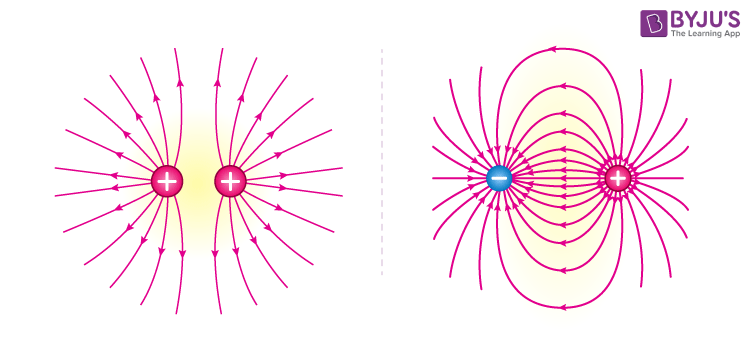# Electric Field Lines

## What is Electric Field Line?

Electric field lines are an excellent way of visualizing electric fields. They were first introduced by Michael Faraday himself.

A field line is drawn tangential to the net at a point. Thus at any point, the tangent to the electric field line matches the direction of the electric field at that point. Secondly, the relative density of field lines around a point corresponds to the relative strength (magnitude) of the electric field at that point. In other words, if you see more electric field lines in the vicinity of point A as compared to point B, then the electric field is stronger at point A.

## Properties of Electric Field Lines

• The field lines never intersect each other.
• The field lines are perpendicular to the surface of the charge.
• The magnitude of charge and the number of field lines, both are proportional to each other.
• The start point of the field lines is at the positive charge and end at the negative charge.
• For the field lines to either start or end at infinity, a single charge must be used.

### Electric Field Lines Attraction and Repulsion

Electric field lines always point away from a positive charge and towards a negative point. In fact, electric fields originate at a positive charge and terminate at a negative charge.Also, field lines never cross each other. If they do, it implies that there are two directions for the electric field at that point. But this is impossible since electric fields add up vectorially at any point and remember that “A field line is drawn tangentially to the net electric field at a point”. Thus, electric field lines can never intersect one another.

As said before field lines are a great way to visualize electric fields. You can almost feel the attraction between unlike charges and the repulsion between like charges as though they are trying to push each other away.Coming to our initial example of static charge on hair, the direction in which charged hair stands up traces the local electric field lines. The charges on the hair exert forces on the hair strand as they attempt to leak into the surrounding uncharged space. The hair aligns accordingly so that there is no net force acting on it and inadvertently traces the electric field lines.

### Rules for Drawing Electric Field Lines

Following are the rules for drawing electric field lines:

1. The field line begins at the charge and ends either at the charge or at infinity.
2. When the field is stronger, the field lines are closer to each other.
3. The number of field lines depends on the charge.
4. The field lines should never crossover.
5. Electric field and electric field line are tangent at the point where they pass through.

#### Recommended Video:## Frequently Asked Questions – FAQs

Q1

### What is an electric field line?

Electric field lines are an excellent way of visualising electric fields. They were first introduced by Michael Faraday himself. A field line is drawn tangentially to the net at a point. Thus at any point, the tangent to the electric field line matches the direction of the electric field at that point. Secondly, the relative density of field lines around a point corresponds to the relative strength (magnitude) of the electric field at that point.
Q2

### What are the properties of electric field lines?

The field lines never intersect each other.
• The field lines are perpendicular to the surface of the charge.
• The magnitude of charge and the number of field lines, both are proportional to each other.
• The start point of the field lines is at the positive charge and ends at the negative charge.
• For the field lines to either start or end at infinity, a single charge must be used.
Q3

### What are the rules for drawing electric field lines?

Following are the rules for drawing electric field lines:
1. The field line begins at the charge and ends either at the charge or at infinity.
2. When the field is stronger, the field lines are closer to each other.
3. The number of field lines depends on the charge.
4. The field lines should never crossover.
5. Electric field and electric field line are tangent at the point where they pass through.
Q4

### What is an electric charge?

Electric Charge is the property of subatomic particles that causes it to experience a force when placed in an electric and magnetic field.
Q5

### State Coulomb’s law.

The magnitude of the electrostatic force of attraction or repulsion between two point charges is directly proportional to the product of the magnitudes of charges and inversely proportional to the square of the distance between them.
Test Your Knowledge On Electric Field Lines!